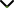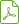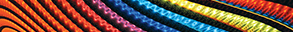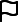# Analytical and Numerical Methods for Solving Partial Differential Equations and Integral Equations Arising in Physical Models

Publishing date
25 Oct 2013
Status
Published
07 Jun 2013

Guest Editors

1Department of Mathematics, National Institute of Technology, Rourkela, India

2Department of Mechanical Engineering, Southern Illinois University, Carbondale, IL, USA

3Department of Science, National Institute of Technical Teachers’ Training and Research, Kolkata, India

4Bhaba Atomic Research Centre, Trombay, Mumbai, India

# Analytical and Numerical Methods for Solving Partial Differential Equations and Integral Equations Arising in Physical Models

## DescriptionPartial differential equations (PDEs) have become a useful tool for describing the natural phenomena of science and engineering models. Nowadays, the most of the phenomena that arise in mathematical physics and engineering fields can be described by PDEs. Many engineering applications are simulated mathematically as PDEs with initial and boundary conditions. Most physical phenomena of fluid dynamics, quantum mechanics, electricity, and many other models are controlled within their domain of validity by PDEs. Therefore, it becomes increasingly important to be familiar with all traditional and recently developed methods for solving PDEs and the implementations of these methods.

For many years the subject of functional equations has held a prominent place in the attention of mathematicians. In more recent years this attention has been directed to a particular kind of functional equation, an integral equation, where in the unknown function occurs under the integral sign. Such equations occur widely in diverse areas of applied mathematics and physics. They offer a powerful technique for solving a variety of practical problems. One obvious reason for using the integral equation rather than differential equations is that all of the conditions specifying the initial value problems or boundary value problems for a differential equation can often be condensed into a single integral equation. Whether one is looking for an exact solution to a given problem or having to settle for an approximation to it, an integral equation formulation can often provide a useful way forward. For this reason integral equations have attracted attention for most of the last century.

This special issue is intended to present recent trends and advances of analytical and numerical methods for the solutions of partial differential equations and integral equations arising in physical models. Potential topics include, but are not limited to:

• Recent developments of partial differential equation models in the real physical systems
• Mathematical modeling of integral equations in physical systems
• New reliable analytical and numerical methods for the solution of partial differential and integral equations
• Advances and applications of partial derivatives and integral equations in mechanics, electricity, economics, finance, biology, control theory, nonlinear waves, and chaos systems

Before submission authors should carefully read over the journal’s Author Guidelines, which are located at http://www.hindawi.com/journals/aaa/guidelines/. Prospective authors should submit an electronic copy of their complete manuscript through the journal Manuscript Tracking System at http://mts.hindawi.com/submit/journals/aaa/spde/ according to the following timetable:Download Special IssueJournal metrics
Acceptance rate14%
Submission to final decision40 days
Acceptance to publication54 days
CiteScore1.300
Impact Factor-Author guidelinesEditorial boardDatabases and indexingSign up for content alerts

We are committed to sharing findings related to COVID-19 as quickly as possible. We will be providing unlimited waivers of publication charges for accepted research articles as well as case reports and case series related to COVID-19. Review articles are excluded from this waiver policy. Sign up here as a reviewer to help fast-track new submissions.